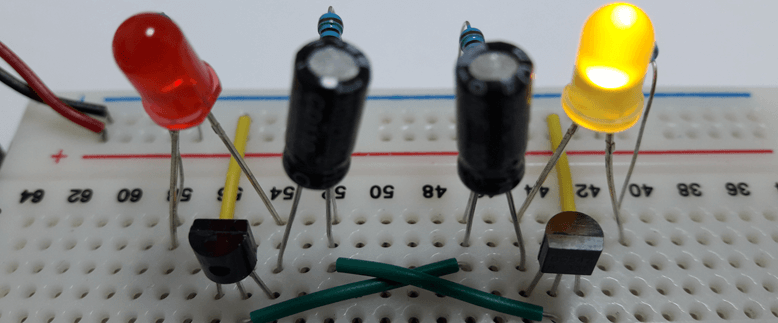# Flip Flop LED Flasher Using BC547 Transistors

This post presents circuit for blinking two LEDs alternatively using two BC547 transistors. This circuit is commonly called a flip flop LED flasher. Generally used in alarm systems to produce flashing lights effect.

## Circuit diagram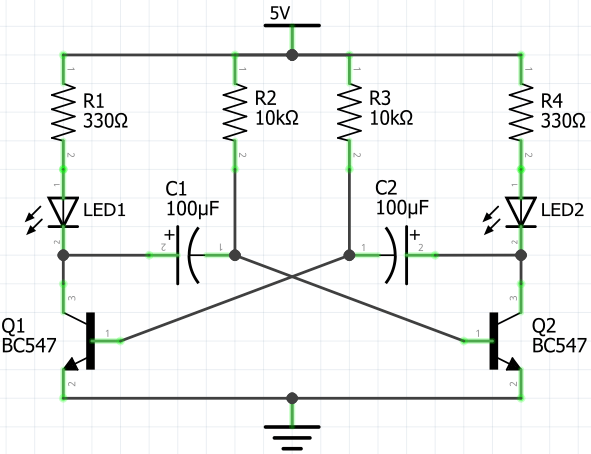The blinking rate of the LEDs can be increased or decreased by changing the values of R2, R3 resistors, and C1, C2 capacitors. The frequency of a flashing LEDs can be calculated by given formula: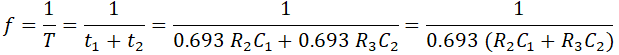If R2, R3 resistors (R2=R3=R) and C1, C2 capacitors (C1=C2=C) have identical values then frequency can be calculated as follows: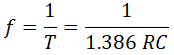The values of resistors are specified in ohms (Ω), values of capacitors are specified in farads (F).

By using values given in the circuit diagram (R2=R3=10kΩ and C1=C2=100μF), the frequency is about 0.72Hz and a time period is 1.386 seconds.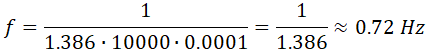## Designed circuit

Circuit is designed on a breadboard.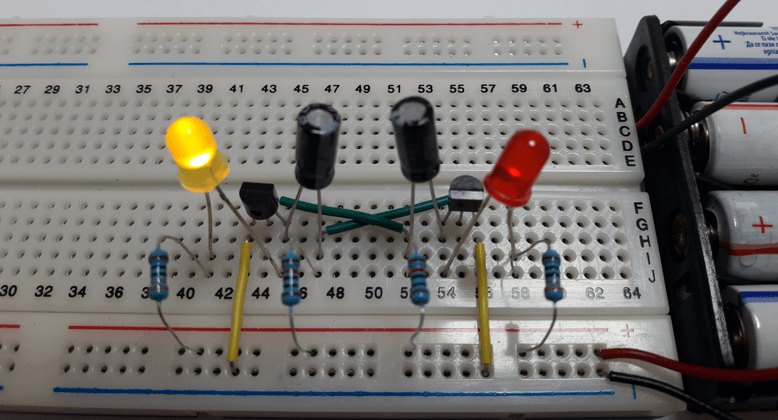1.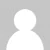halloo I Hope you are doing well I just wanted to conform that how to set the values of Resister R3 R2 or the capacitor c1 c2 to set the blinked frequency to 4 hz or set the time period to 0.25s

•Hi, Tahir Bangash
I updated the post description. I provided formulas and calculation example. You can choose 18kΩ R2, R3 resistors and 10μF C1, C2 capacitors. The frequency approximately will be 4Hz and a time period will be about 0.25 seconds.
`f = 1 / (1.386 * 18000 * 0.00001) ≈ 1 / 0.25 ≈ 4Hz`

2.Hi guys,
I am a beginner in electronics, and wanted to make this circuit. But, after making it it flashed twice and stopped. I tried it again but nothing happened. Can someone with better knowledge of this kind of stuff please help?

Materials used:
BC547 transistor
440Ω resistors
10kΩ resistors
100μF electrolytic capacitors
Green LEDs
Jumper Wires

•Hi
Perhaps your voltage is too low. What your power supply voltage? Try to use 9V battery or change current limiting 440Ω resistors R1 and R4 to lower value, such 330Ω.

•Well if it already flashed twice, so that might not be it. Also I don't have any 330Ω resistors and the output voltage is at 5v. But of course you might be right.

3.Thank you!!!! I finally got this!

4.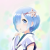Hi, what values need to be changed if I used a 9v power supply?

•Hi,
To use a 9V power supply, you should change current limiting resistors R1 and R4 to higher value, such 470Ω.

•